• 网上查到的，转载下，已测试可以使用 ...h=bar(x,1,'group'); set(gca,'xticklabels',{'benchmark1','benchmark2','benchmark3','benchmark4','benchmark5'}); h = gca; th=rotateticklabel(h, 45);%
网上查到的，转载下，已测试可以使用

示例：

x = round(rand(5,3)*10);

h=bar(x,1,'group');

set(gca,'xticklabels',{'benchmark1','benchmark2','benchmark3','benchmark4','benchmark5'});

h = gca;

th=rotateticklabel(h, 45);%调用下面的函数，坐标倾斜45度

代码如下：

function th=rotateticklabel(h,rot,demo)
%ROTATETICKLABEL rotates tick labels
%   TH=ROTATETICKLABEL(H,ROT) ris the calling form where H is a handle to
%   the axis that contains the XTickLabels that are to be rotated. ROT is
%   an optional parameter that specifies the angle of rotation. The default
%   angle is 90. TH is a handle to the text objects created. For long
%   strings such as those produced by datetick, you may have to adjust the
%   position of the axes so the labels don't get cut off.
%
%   Of course, GCA can be substituted for H if desired.
%
%   TH=ROTATETICKLABEL([],[],'demo') shows a demo figure.
%
%   Known deficiencies: if tick labels are raised to a power, the power
%   will be lost after rotation.
%
%   Written Oct 14, 2005 by Andy Bliss
%   Copyright 2005 by Andy Bliss
%DEMO:
if nargin==3
x=[now-.7 now-.3 now];
y=[20 35 15];
figure
plot(x,y,'.-')
datetick('x',0,'keepticks')
h=gca;
set(h,'position',[0.13 0.35 0.775 0.55])
rot=90;
end
%set the default rotation if user doesn't specify
if nargin==1
rot=90;
end
%make sure the rotation is in the range
% 0:360 (brute force method)
% while rot>360
%     rot=rot-360;
% end
% while rot<0
%     rot=rot+360;
% end
%get current tick labels
a=get(h,'XTickLabel');
%erase current tick labels from figure
set(h,'XTickLabel',[]);
%get tick label positions
b=get(h,'XTick');
c=get(h,'YTick');
%make new tick labels
if rot<180
th=text(b,repmat(c(1)-.1*(c(2)-c(1)),length(b),1),a,'HorizontalAlignment','right','fontsize',14,'fontweight','bold','rotation',rot);
else
th=text(b,repmat(c(1)-.1*(c(2)-c(1)),length(b),1),a,'HorizontalAlignment','left','fontsize',14,'fontweight','bold','rotation',rot);
end


展开全文• pcie_debug 用于读取/写入 PCIe BARx 内存空间的命令行工具 链接 该工具源自 DW Hawkins ( )。 原始来源可以在上找到。C
• 画直方时，需将bar放置在坐标轴标值的中间，需要对bins以及x轴标值进行设置，将bin对应的个数及值事先定义好，然后将坐标标值设置为两个bin的中间值，再设计参数align='mid'即可，如：import numpy as np ...
画直方图时，需将bar放置在坐标轴标值的中间，需要对bins以及x轴标值进行设置，将bin对应的个数及值事先定义好，然后将坐标标值设置为两个bin的中间值，再设计参数align='mid'即可，如：

import numpy as np
import os
import matplotlib.pyplot as plt
weights=np.ones_like(area)/float(len(area))
#print np.min(area),np.max(area)  bins=np.arange(0,np.max(area)+40,25)-12.5#print bins plt.hist(area,bins=bins,weights=weights,rwidth=barwidth,facecolor='blue',alpha=0.75,align='mid') plt.xticks(range(0,560,50)) plt.xlim([-25,550]) plt.title('Area > 10mm of North China Warm Sector rainfall') plt.xlabel('Area > 10mm') plt.ylabel('Probability') plt.savefig('D:\\research\\pics\\statistic_warmsectorcases\\NorthChinaBasin_warm_sector_Area.png') plt.close()
参考 https://stackoverflow.com/questions/27083051/matplotlib-xticks-not-lining-up-with-histogram


展开全文• 基于现有的 M 文件 errorbar.m，进行了修改，以便在水平方向绘制误差线。 此外，该文件考虑 X 或 Y 向量中的单个值。此 M 文件可用于绘制粉末粒度分布的 D_50 值中的分布。matlab
• ECharts 统计中的bar（条形统计）的X轴上的值的显示方法


ECharts 框架的 bar 统计图
ECharts 统计图中的bar（条形统计）的X轴上的值的显示方法一共有三种，先上图：
第一种：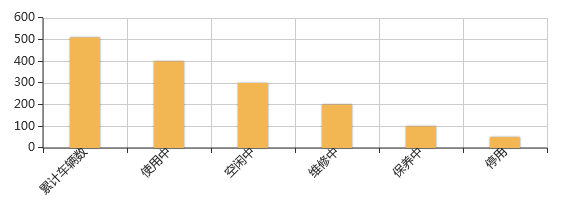第二种：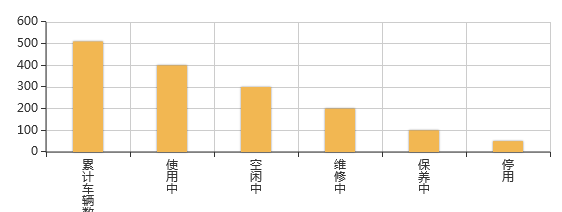第三种：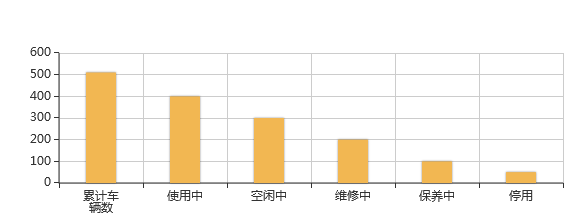以下是源码：

<!DOCTYPE html>
<html>
<meta charset="utf-8">
<!-- 引入 ECharts 文件 -->
<script src="../js/echarts.min.js"></script>
<script src="../js/newline-echarts.js"></script>

<body>
<!-- 为 ECharts 准备一个具备大小（宽高）的Dom -->
<div id="main" style="width: 100%;height:250px;"></div>
<script type="text/javascript">
// 基于准备好的dom，初始化echarts实例
var myChart = echarts.init(document.getElementById('main'));

// 指定图表的配置项和数据
var option = {
barWidth:30,//设置 柱状图的宽度为30px 如果不设置barWidth属性 宽度会自动变化 ;
tooltip: {},

xAxis: {
type : 'category',
axisLabel:{
//横坐标上的文字斜着显示 begin
interval:0,
rotate:45,
margin:2,
textStyle:{
color:"#222"
}
//横坐标上的文字斜着显示 end
//横坐标上的文字换行显示 begin
/* formatter:function(val){
return val.split("").join("\n");
}*/
//横坐标上的文字换行显示 end
},
data: ["累计车辆数","使用中","空闲中","维修中","保养中","停用"]
},
yAxis: {},
series: [{

type: 'bar',
data: [510, 400, 300, 200, 100, 50],
itemStyle: {
normal: {
color: '#F2B752',
}
}
}]/*,
grid: { // 控制图的大小，调整下面这些值就可以，
x: 40,
x2: 100,
y2: 150,// y2可以控制 X轴跟Zoom控件之间的间隔，避免以为倾斜后造成 label重叠到zoom上
},*/
};

// 使用刚指定的配置项和数据显示图表。
//X轴上的值显示不完 3个文字就换行显示 引用newline-echarts.js JS
/*option= newline(option, 3, 'xAxis');*/
myChart.setOption(option);
</script>
</body>
</html>

当X轴（横坐标）的值显示不完整是 可以使用一下三个方法解决
第一种：字体斜着显示
axisLabel:{                    //横坐标上的文字斜着显示 begin
interval:0,
rotate:45,
margin:2,
textStyle:{
color:"#222"
}

//横坐标上的文字斜着显示 end
第二种：字体竖着显示
axisLabel:{
//横坐标上的文字换行显示 begin                      formatter:function(val){                        return val.split("").join("\n");                    }
//横坐标上的文字换行显示 end}

第三种：引用newline-echarts.js文件
//X轴上的值显示不完  3个文字就换行显示 引用newline-echarts.js JS 也可以用在Y轴上        option= newline(option, 3, 'xAxis');
myChart.setOption(option);

展开全文• 设置旋转90度： plt.xticks(rotation=90,fontsize=13) ...plt.bar(range(len(x)), y, color = 'g') 实例代码： # 设置数据 x = np.array(top10['景点名称']) y = np.array(top10['综合得分'...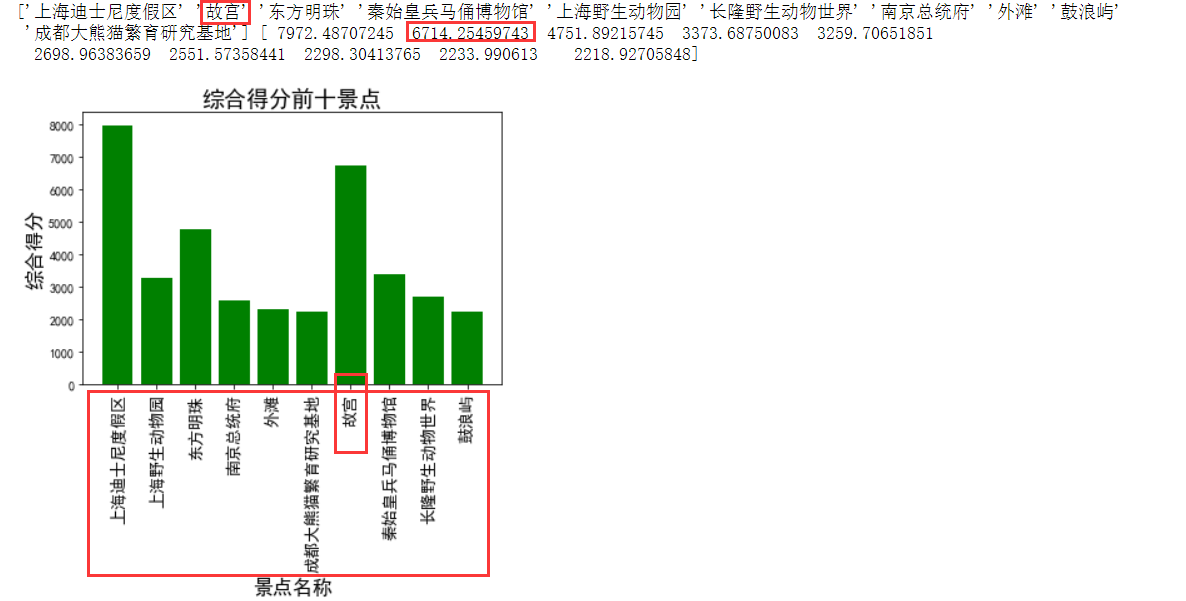设置旋转90度：

plt.xticks(rotation=90,fontsize=13)

调整顺序：

plt.xticks(range(len(x)), x)
plt.bar(range(len(x)), y, color = 'g')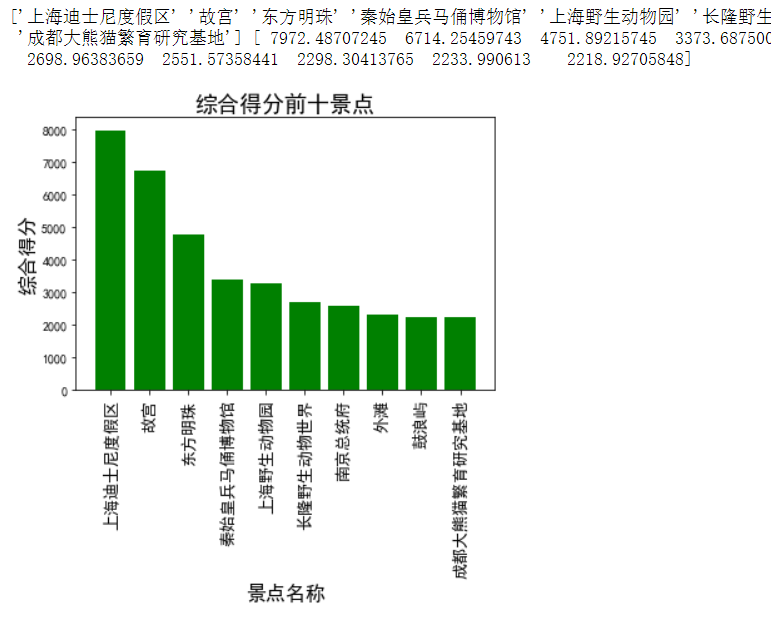实例代码：
# 设置数据
x = np.array(top10['景点名称'])
y = np.array(top10['综合得分'])
print(x, y)
plt.xticks(range(len(x)), x)
# 绘图
plt.bar(range(len(x)), y, color = 'g')
plt.xlabel('景点名称', fontsize=16)
plt.xticks(rotation=90,fontsize=13)
plt.ylabel('综合得分', fontsize=16)
plt.title('综合得分前十景点', fontsize=18)
plt.savefig('./image/综合得分前十景点.png')
plt.show()

展开全文• 苹果2017款带触摸条笔记本电脑维修专用原理、电路、点位。APPLE MACBOOK PRO TOUCH BAR X362 MLB 820-00239.pdf
• 代码如下 import numpy as np import matplotlib.pyplot as plt N = 5 y = [20,10,30,25,15] ... pl = plt.bar(left=index... TypeError: bar() missing 1 required positional argument: 'x'matplotlib
• 使用matlab补丁进行实施，并使用默认的颜色映射。 使用 colormap() 从函数外部更改颜色。matlab
• bar3”和“hist3”都不允许从一行到下一行宽度变化的列； “bar3d”确实如此。 我用它在不规则网格上绘制二维离散分布。matlab
• ## 关于28379D的X-BAR

千次阅读 2020-02-11 20:09:16
1. 什么是X-BAR？ 来自TI官方文档： The crossbars (referred to as X-BAR throughout this document) provide flexibility to connect device inputs, outputs, and internal resources in a variety of ...
• 在matlab中，可以使用bar3（）函数来解决三维的柱状绘制问题，假设有下面的代码，得到图像为： a = [1,1,1;2,2,2;3,3,3] bar3(a); 二、bar3(Y,Z)的用法 若在上述代码的前提下，想调整生成的柱状的横轴，...matlab set函数
• 问题描述使用matlibplot.pyplot中的axes.bar()作图后x轴是默认排序的，这其实打乱了我一开始数据中的排序，是我不想要的，我想要的是x轴按照我数据中的原始顺序排序。 import matplotlib.pyplot as plt In :...matlibplot plot Python
• 现在通过与AndroidX兼容 Flutter Statusbar Manager，可让您控制iOS和Android上的状态栏颜色，样式（主题），可见性和半透明属性。 并为Android提供了一些额外的奖励来控制导航栏。 这个插件基于React Native的...
• 有關CPK的知識及X-bar,有關CPK的知識及X-bar
• 在pandas dataframe中使用plot画图，当x轴是日期的时候，x轴会显示时间和分钟。如果使用set_major_formatter函数格式化ax.xaxis.set_major_formatter(DateFormatter('%Y-%m-%d'));那么会报错ValueError: ...
• 使用touchbar来控制飘落emoji表情雨。 开源应用，只为你玩的开心。 该版本为Mac版本，双击app文件直接运行，源代码请移步网站：https://github.com/KaiOrange/touchbar-emoji
• 我看中了用 bar 函数绘制直方， 具体函数使用方法，参考帮助文档，此处讨论直方的控制 目标，实现 改x坐标 实际是设置Axes属性，查帮助，设置XTickLabel 。 ax = gca; ax.XTick = 1: 5; ax.XTickLabel
•C2000
• ## Matlab bar绘制条形图

千次阅读 2015-01-26 19:33:48
MATLAB中绘制条形的方法比较简单，给定样本值，直接调用bar()函数即可完成基本的条形的绘制，bar3()可以绘制三维条形。  bar()函数可以直接使用bar(y)绘制，这时的x从1开始递增；或者与plot(x,y)函数方法...
• 在matplotlib中，bar命令用于绘制柱状，基本用法如下在matplotlib中，bar命令用于绘制柱状，基本用法如下在matplotlib中，bar命令用于绘制柱状，基本用法如下在matplotlib中，bar命令用于绘制柱状，基本用法...
• bar(x, height, width=0.8, bottom=None, ***, align='center', data=None, **kwargs) x 表示x坐标，数据类型为int或float类型， height 表示柱状的高度，也就是y坐标值，数据类型为int或float类型， width 表示...python 详解
• VueJS 2x的虚拟响应式跨浏览器滚动条组件 用法 安装 使用NPM npm i v-bar 使用纱 yarn add v-bar 在您的.vue组件中，为包装器创建一个类 使用SASS < style lang = " sass " > .wrapper height : 300 px ...
• X HOME BAR 1.3.0_sign_20180215170435安卓全面屏幕手势汉化版，x HOME bar不是一般的好用，完美隐藏虚拟键。是那种永久隐藏，手势代替虚拟按键全面屏手势
• 用chart.js做横向柱状图表（horizontalBar）时，通常是下面这个样子的： 默认情况下，X轴（数值刻度）在下方显示。 但有时候，我们可能希望X轴在上方显示，例如，如果Y轴的标签比较多的时候，如果X轴在下方显示的话...js
• 前一段时间作者在进行毕业设计时使用MATLAB绘制了论文中的一系列插图（包括曲线、柱状等），自我感觉其确实是一个必不可少的实用性工具，所以在这里为大家简单介绍一下MATLAB中的基本绘图命令。 plot函数 plot...MATLAB plot
• 【Matlab开发】matlab中bar绘图设置与各种距离度量标签（空格分隔）： 【Matlab开发】 【机器学习】声明：引用请注明出处http://blog.csdn.net/lg1259156776/Matlab Bar图如何为每个bar设置不同颜色data = [3, 7, 5,...matlab 开发 机器学习
• #a = plt.bar(np.arange(len(x_sort)),y_sort,color=['r','g','b', 'c', 'm', 'y'])#这是设置颜色的 #autolabel(a) plt.title('Confindence') plt.ylabel('Confindence-AVG', fontsize=12) plt.xlabel('label', ...nlp
• matlab开发-plotbar3颜色指示sxyz。三维条形，将条形高度编码为颜色...